Question

You connect three capacitors as shown in the diagram below. If the potential difference between A...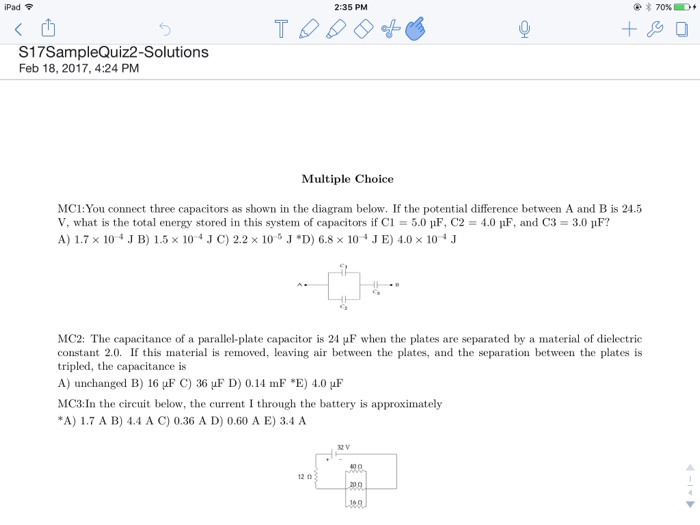You connect three capacitors as shown in the diagram below. If the potential difference between A and B is 24.5 V. what is the total energy stored in this system of capacitors if C1 = 5.0 mu F, C2 = 1.0 mu F, and C3 = 3.0 mu F? 1.7 times 10^-4 J. 1.5 times 10^-4 J 2.2 times 10^-5 J 6.8 times 10^-4 J 4.0 times 106-4 J The capacitance of a parallel-plate capacitor is 21 mu F when the plates are separated by a material of dielectric constant 2.0. If this material is removed, leaving air between the plates, and the separation between the plates is tripled, the capacitance is unchanged 16 mu F 36 mu F 0.11 mu F 4.0 mu F In the circuit below, the current I through the battery Ls approximately 1.7 A 4.4 A 0.36 A 0.60 A 3.4 A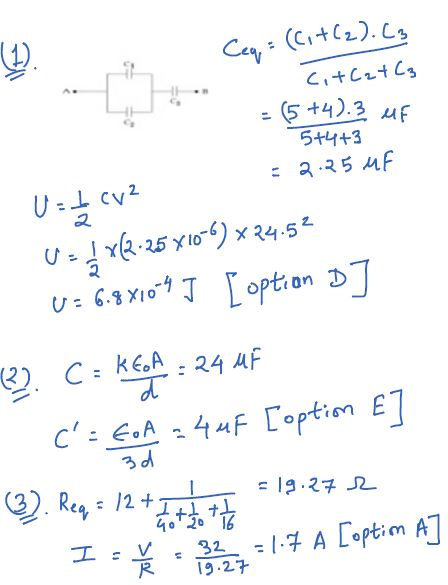Earn Coins

Coins can be redeemed for fabulous gifts.

Similar Homework Help Questions
• A battery V=15 V is connected to three Capacitors in the configuration shown below, The Capacitance...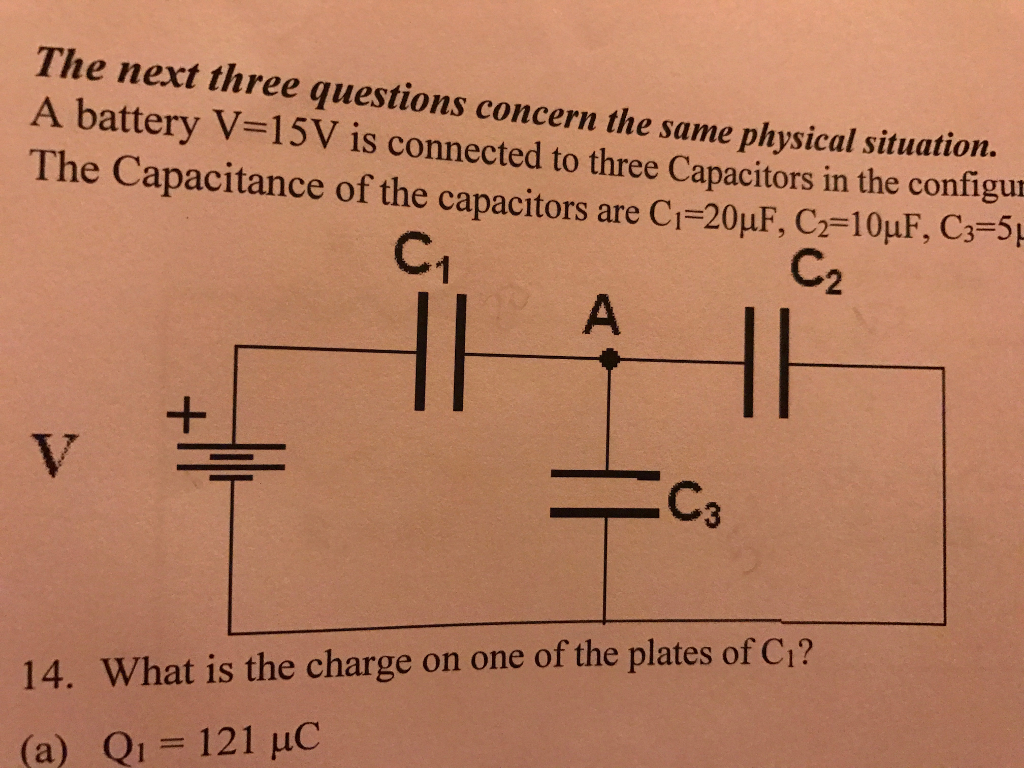A battery V=15 V is connected to three Capacitors in the configuration shown below, The Capacitance of the Capacitors are C1= 20 uF, C2= 10uF and C3=5 uF. 2. Compute the total stored energy in all of the capacitors. a. Utot= 2.5*10^-4 J b. Utot= 6.4*10^-4 J c. Utot= 9.6*10^-4 J d. Utot= 4.1*10^-4 J e. Utot= 6.0*10^-4 J

• Part A and Part B please Part A Constants What is the potential difference between the...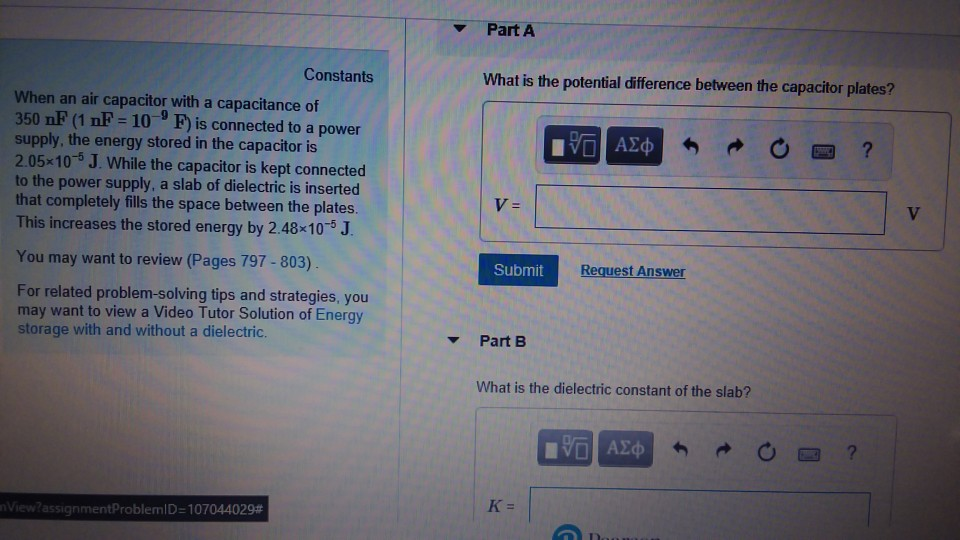Part A and Part B please Part A Constants What is the potential difference between the capacitor plates? When an air capacitor with a capacitance of 350 nF (1 nF 10 F is connected to a power supply, the energy stored in the capacitor is 2.05x10-s J. While the capacitor is kept connected to the power supply, a slab of dielectric is inserted that completely fills the space between the plates. This increases the stored energy by 2.48x105 J. You...

• Problem 5 In the figure below C1 2.0 μF, G 10.0pE, C3 3,0 μF , C.-6.0 μF.G-4.0 μ. The applied potential difference between a and b is Vab 10V. a) [4 points] Calculate the equivalent capacitance o...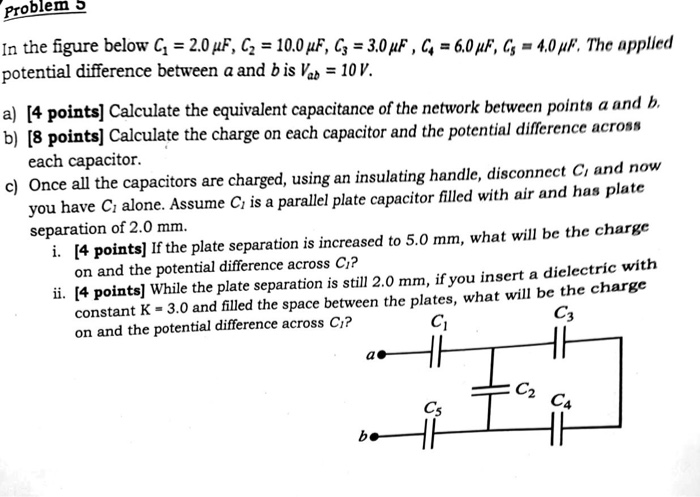Problem 5 In the figure below C1 2.0 μF, G 10.0pE, C3 3,0 μF , C.-6.0 μF.G-4.0 μ. The applied potential difference between a and b is Vab 10V. a) [4 points] Calculate the equivalent capacitance of the network between points a and b. b) [8 pointsl Calculate the charge on each capacitor and the potential difference across each capacitor. c) Once all the capacitors are charged, using an insulating handle, disconnect Ci and now you have C alone. Assume...

• capacitors are connected as shown to a potential difference of V=14.0 volts. CisC,-Cr=C,-C,-20 μ1: The charge...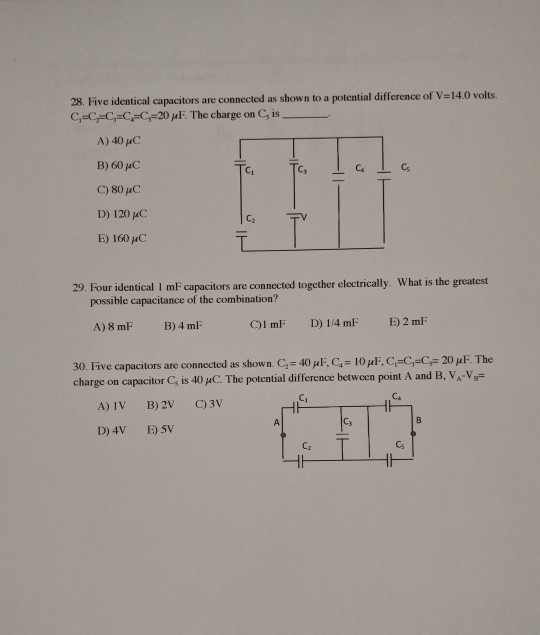capacitors are connected as shown to a potential difference of V=14.0 volts. CisC,-Cr=C,-C,-20 μ1: The charge on C5 is A) 40 pC B) 60 HC C) 80 uC D) 120 HC E) 160 μC C1 C3 C2 29·Four identical 1 mF capacitors are connected together electrically what is the greatest possible capacitance of the combination? A) 8 mF B) 4 mF 30 Five capacitors are connected as shown. C2 40 μ F, C4# 10 μ1, Ci=C,sCF 20 μ F The...

• Problems 4-9 refer to this same capacitor: The plates of a parallel plate capacitor each have...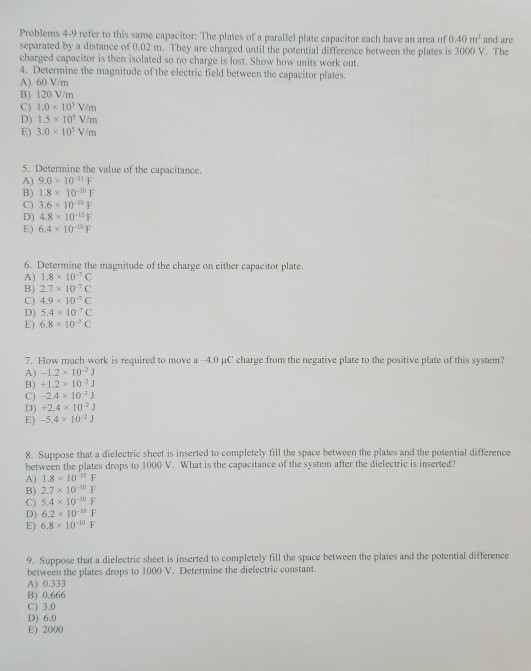Problems 4-9 refer to this same capacitor: The plates of a parallel plate capacitor each have an area of 0.40 m and are separated by a distance of 0.02 m. They are charged until the potential difference between the plates is 3000 V. The charged capacitor is then isolated so no charge is lost. Show how units work out. 4. Determine the magnitude of the electric field between the capacitor plates. A) 60 V/m B) 120 V/m C) 1.0 x...

• A circuit is constructed with five capacitors and a battery as shown. The values for the...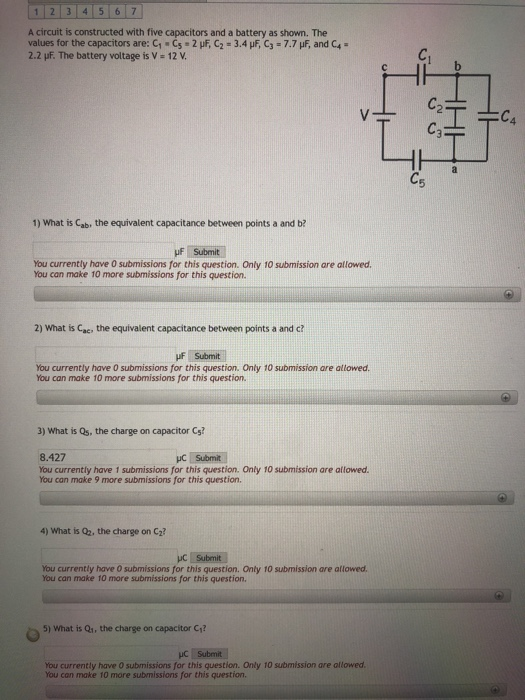A circuit is constructed with five capacitors and a battery as shown. The values for the capacitors are: C1 . С5 . 2 ,F, c,. 3.4 F, C3-77 F, and C+" 2.2 pF. The battery voltage is V 12v 1) What is Cab, the equivalent capacitance between points a and b? uF Submit You currently have O submissions for this question. Only 10 submission are allowed. You can make 10 more submissions for this question. 2) What is ac, the...

• Question-20 5 points Capacitors Cz, C2 and Cz are connected as shown in the circuit below....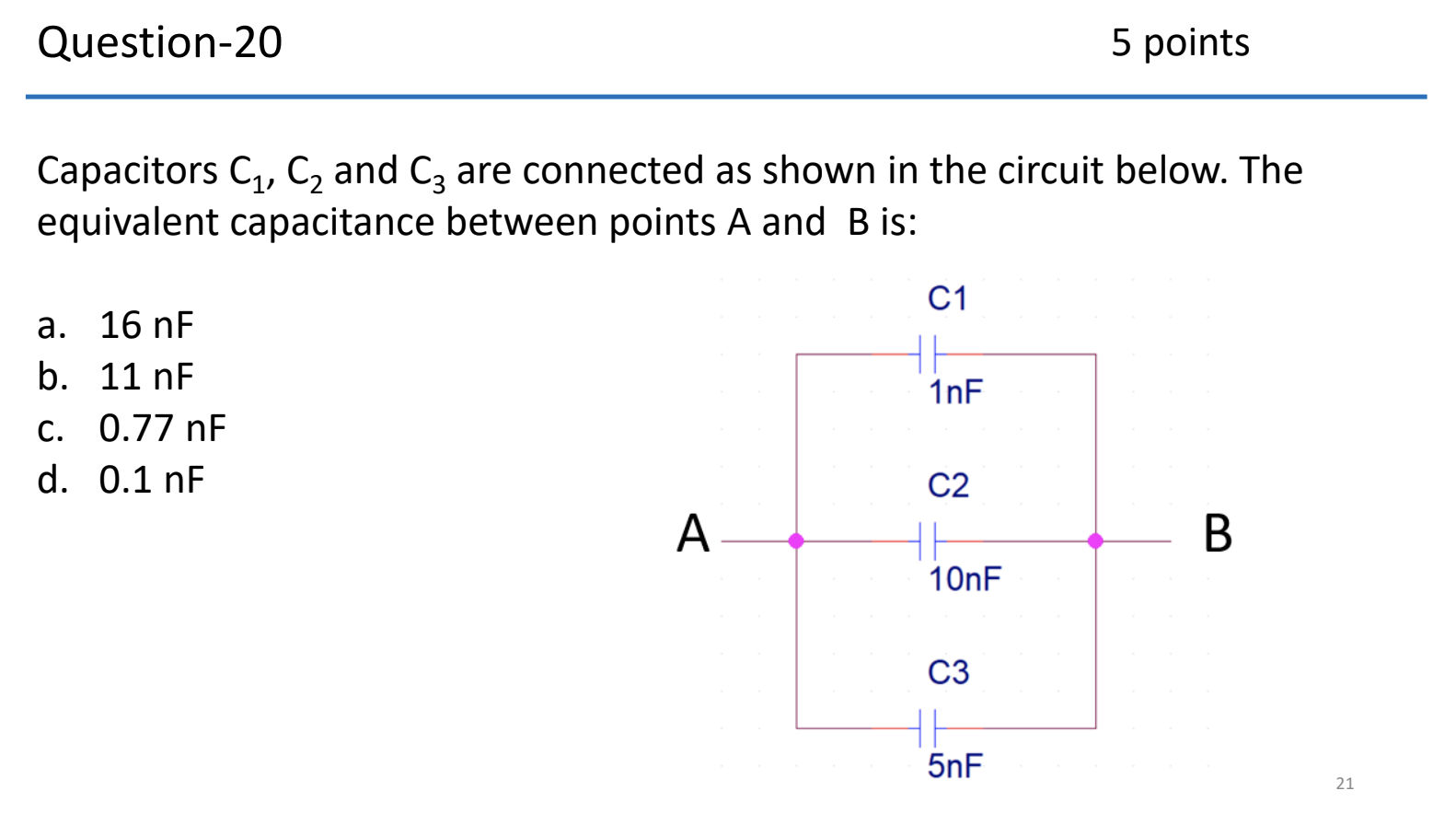Question-20 5 points Capacitors Cz, C2 and Cz are connected as shown in the circuit below. The equivalent capacitance between points A and B is: C1 a. 16 nF b. 11 nF C. 0.77 nF d. 0.1 nF 1nF C2 10nF C3 InF Question-21 5 points The impedance, Z of a 10 mH inductor at a frequency of f = 1000 Hz, expressed as a complex number is a. –j 62.83W b. +j 62.83W C. –j 10W d. +j 10w...

• As shown in the cireuit to the right, a potential difference of 625 V is applied...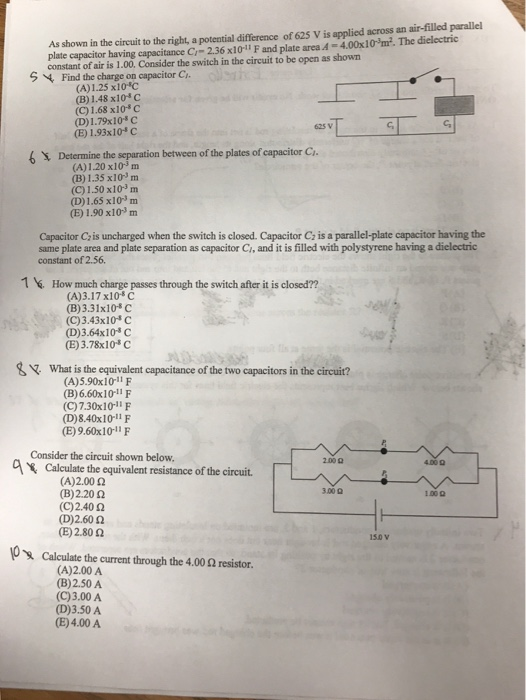As shown in the cireuit to the right, a potential difference of 625 V is applied across an air-filled parallel plate capacitor having capacitance Cr-2.36 x101l F and plate area 4-4.00x10m2. The dielectric constant of air is 1.00, Consider the switch in the circuit to be open as shown S 4 Find the charge on capacitor C (A)1.25 x10*C (B) 1.48 x10 c (C) 1.68x10 (D) 1.73x10-C (E) 1.93x10*c 625 v G, 6 Determine the between of the plates of...

• al Figure E1.1 Capacitor network or the system of capacitors shown in Figure E 1.1, C1-10.0...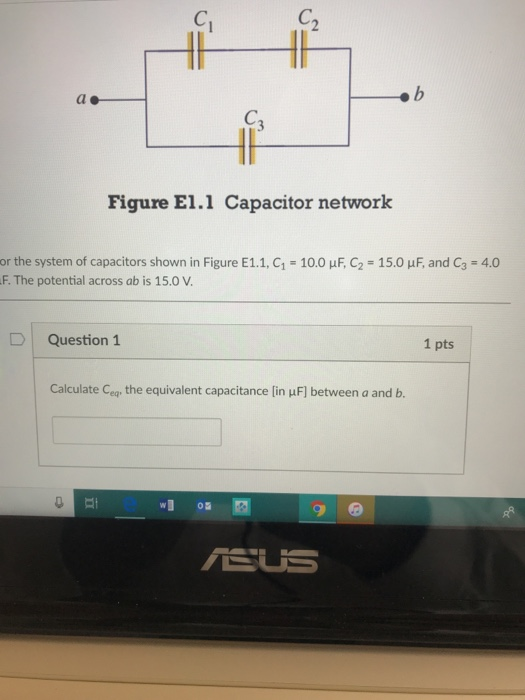al Figure E1.1 Capacitor network or the system of capacitors shown in Figure E 1.1, C1-10.0 μΕ C2-15.0 μΕ and C3-4.0 F. The potential across ab is 15.0 V Question1 1 pts Calculate Ceq the equivalent capacitance [in uF] between a and b. wo ELE Calculate Q, the total energy lin HJ] stored on the system of capacitors. Question 3 1 pts Calculate Q, the energy [in W] stored on capacitor C1. D | Question 4 1 pts Calculate Q2,...

• v3. At what distance from a point charge of 4.0 nC does the electric field have...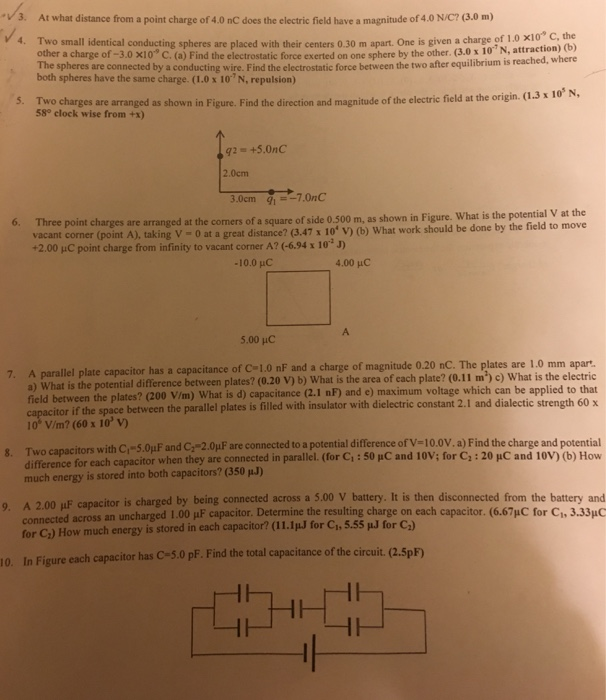v3. At what distance from a point charge of 4.0 nC does the electric field have a magnitude of 4.0 N/C? (3.0 m) 4. Two small identical conducting spheres are placed with their centers 0.30 m apart. One is given a charge of 1 0 x10* c other a charge of -3.0 x10 C. (a) Find the electrostatic force exerted on one sphere by the other. (3.0 x 10"N, attraction) the (b) e spheres are connected by a conducting wire....